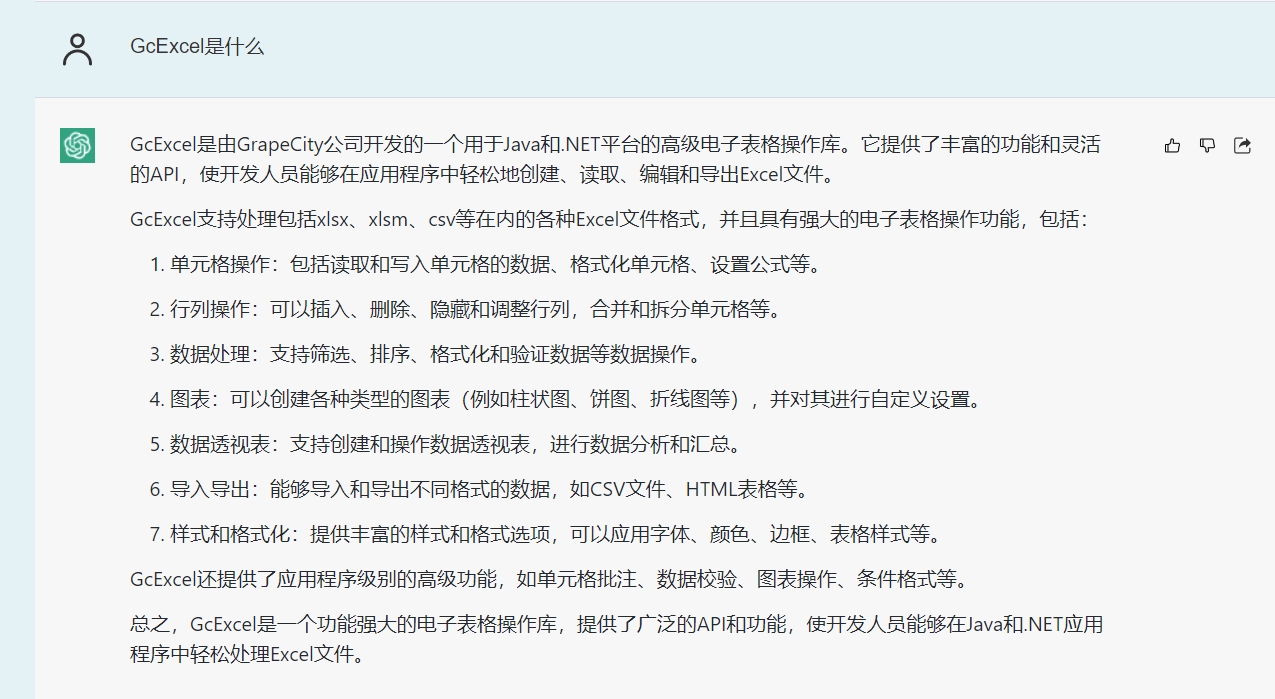07/05 15:33

# 前言 | 问题背景### 场景1 批量导入Excel文件，并读取特定区域的数据@Test

public void testImportFormFile() {

String folderPath = "path/testFolder"; //使用你的路径

File folder = new File(folderPath);

File[] files = folder.listFiles();

if (files != null) {

for (File file: files){

if(file.isFile() && file.getName().endsWith(".xlsx")){

Workbook wb = new Workbook();

wb.open(file.getAbsolutePath());

Object[][] value = (Object[][]) wb.getActiveSheet().getRange("B3:C6").getValue();

System.out.println(value); //小葡萄

System.out.println(value); //20.0

System.out.println(value); //开发部

System.out.println(value); //610123456789012345

//添加处理数据的逻辑

}

}

}

}


### 场景2 批量导出Excel文件，导出前把数据写在特定位置

@Test
public void testExportFormFile() {
String outPutPath = "E:/testFolder";
//给valueList初始化数据，替换为从数据库，CSV或者JSON等中获取数据。
ArrayList<Object[][]> valueList = new ArrayList<Object[][]>();
for (Object[][] value : valueList) {
Workbook wb = new Workbook();
wb.getActiveSheet().getRange("B3:C6").setValue(value);
wb.save(outPutPath + UUID.randomUUID().toString() + ".xlsx");
}
}


GcExcel可以直接把二维数组设置给一个range，从数据库中把数据加载出来以后，可以整理成二维数组。

### 场景3 打开Excel文件，批量替换关键字

@Test
public void testReplaceTemplateFile() {
String templateFilePath = "test.xlsx";
Workbook wb = new Workbook();
wb.open(templateFilePath);
IRange usedRange = wb.getActiveSheet().getUsedRange();
ArrayList<Object[]> valueList = new ArrayList<Object[]>();
for (Object[] value : valueList) {
usedRange.replace(value,value);
}
wb.save("result.xlsx", SaveFileFormat.Xlsx);
}


### 场景4 打开Excel模板文件，批量获取计算结果

@Test
public void testCalcFormulaByTemplateFile() {
String templateFilePath = "E:/testFolder/testFormula.xlsx";
Workbook wb = new Workbook();
wb.open(templateFilePath);
//获取特定的值，比如以下
ArrayList<Object[]> valueList = new ArrayList<Object[]>();
for (Object[] value : valueList) {

Object A1Value = value;
Object A2Value = value;
Object result = null;
wb.getActiveSheet().getRange("A1").setValue(A1Value);
wb.getActiveSheet().getRange("A2").setValue(A2Value);
result = wb.getActiveSheet().getRange("A3").getValue();
System.out.println(result);
}
}



GcExcel的公式计算是在取值的时候计算的，因此不需要显示调用calculate之类的方法，只需要把输入的参数准备好，放在Excel特定的cell中，就可以直接获取公式的计算结果了。

GrapeCity Documents for Excel（服务端Excel组件）V3.0 正式发布0 评论
1 收藏
0